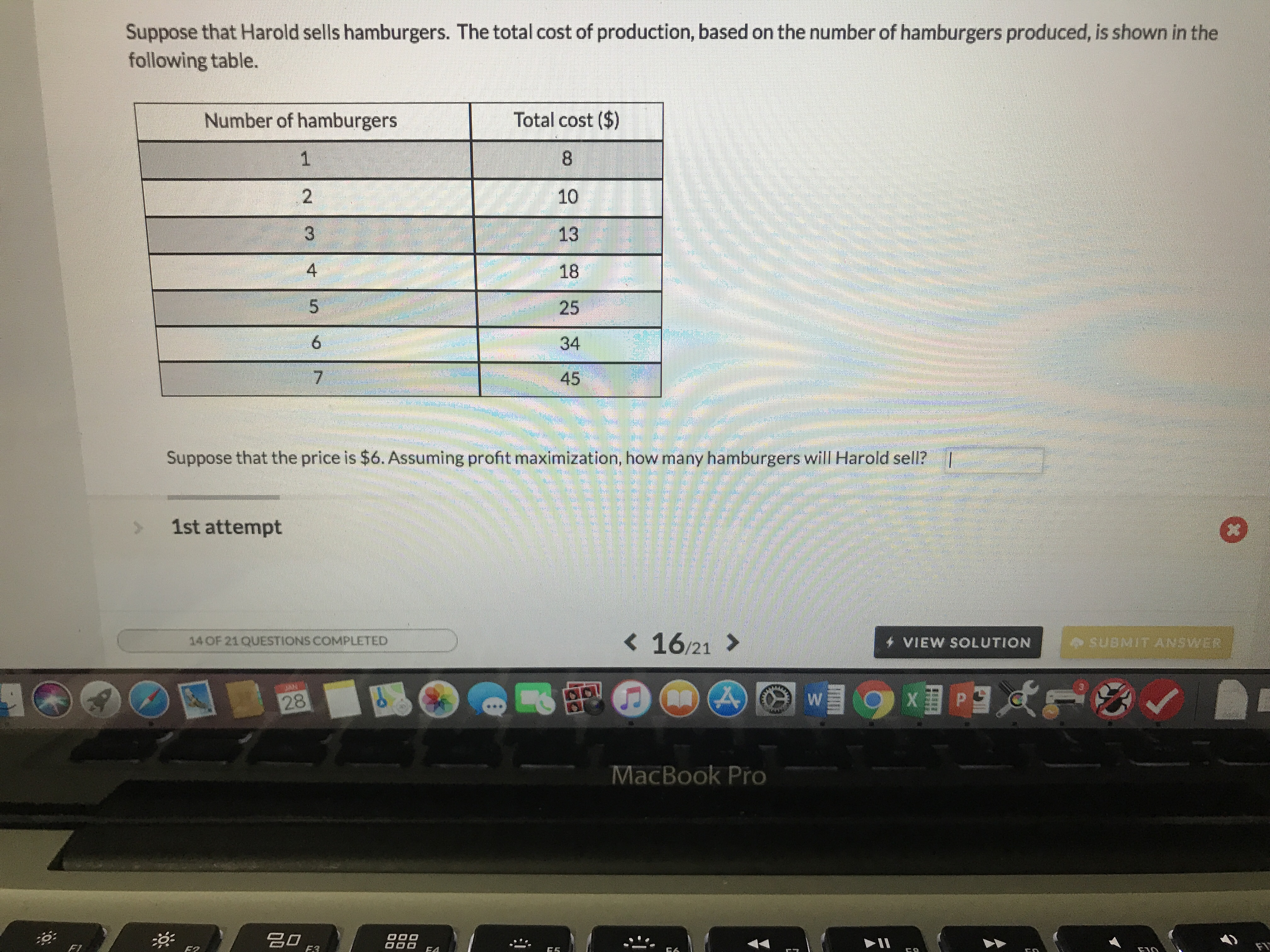# Suppose that Harold sells hamburgers. The total cost of production, based on the number of hamburgers produced, is shown in thefollowing table.Number of hamburgersTotal cost (\$)10131825344524Suppose that the price is \$6. Assuming profit maximization, how many hamburgers will Harold sell?I1st attempt< 1621 >VIEW SOLUTION14 OF 21 QUESTIONS COMPLETEDSUBMIT ANSWER28MacBook Pro漠:名□F2

Question
536 viewshelp_outlineImage TranscriptioncloseSuppose that Harold sells hamburgers. The total cost of production, based on the number of hamburgers produced, is shown in the following table. Number of hamburgers Total cost (\$) 10 13 18 25 34 45 2 4 Suppose that the price is \$6. Assuming profit maximization, how many hamburgers will Harold sell? I 1st attempt < 1621 > VIEW SOLUTION 14 OF 21 QUESTIONS COMPLETED SUBMIT ANSWER 28 MacBook Pro 漠: 名□ F2 fullscreen
check_circle

star
star
star
star
star
1 Rating
Step 1

Profit maximization qty is that qty where marginal revenue is equal to or greater than Marginal cost and after that the marginal cost is rising and higher than marginal revenue.

Therefore,  schedule showing the Marginal cost has been prepared as above.

marginal cost of nth unit = Total cost of nth unit - ...

### Want to see the full answer?

See Solution

#### Want to see this answer and more?

Solutions are written by subject experts who are available 24/7. Questions are typically answered within 1 hour.*

See Solution
*Response times may vary by subject and question.
Tagged in